# Drag (physics)

Drag (physics)
Shape and flow Form
drag
Skin
friction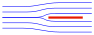0% 100%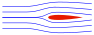~10% ~90%~90% ~10%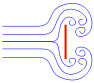100% 0%

In fluid dynamics, drag (sometimes called air resistance or fluid resistance) refers to forces which act on a solid object in the direction of the relative fluid flow velocity.    Unlike other resistive forces such as dry friction, which is nearly independent of velocity, drag forces depend on velocity.

Drag forces always decrease fluid velocity relative to the solid object in the fluid's path.

## Examples of drag

Examples of drag include the component of the net aerodynamic or hydrodynamic force acting opposite to the direction of the movement of the solid object relative to the Earth as for cars, aircraft and boat hulls; or acting in the same geographical direction of motion as the solid, as for a sails on a down wind sail boat, or in intermediate directions on a sail depending on points of sail.    In the case of viscous drag of fluid in a pipe, drag force on the immobile pipe decreases fluid velocity relative to the pipe. 

## Types of drag

Types of drag are generally divided into the following categories:

The phrase parasitic drag is mainly used in aerodynamics, since for lifting wings drag is in general small compared to lift. For flow around bluff bodies, drag is most often dominating, and then the qualifier "parasitic" is meaningless. Form drag, skin friction and interference drag on bluff bodies are not coined as being elements of parasitic drag, but directly as elements of drag.
Further, lift-induced drag is only relevant when wings or a lifting body are present, and is therefore usually discussed either in the aviation perspective of drag, or in the design of either semi-planing or planing hulls. Wave drag occurs when a solid object is moving through a fluid at or near the speed of sound in that fluid—or in case there is a freely-moving fluid surface with surface waves radiating from the object, e.g. from a ship.

For high velocities—or more precisely, at high Reynolds numbers—the overall drag of an object is characterized by a dimensionless number called the drag coefficient, and is calculated using the drag equation. Assuming a more-or-less constant drag coefficient, drag will vary as the square of velocity. Thus, the resultant power needed to overcome this drag will vary as the cube of velocity. The standard equation for drag is one half the coefficient of drag multiplied by the fluid mass density, the cross sectional area of the specified item, and the square of the velocity.

Wind resistance is a layman's term used to describe drag. Its use is often vague, and is usually used in a relative sense (e.g., a badminton shuttlecock has more wind resistance than a squash ball).

## Drag at high velocityExplanation of drag by NASA.

The drag equation calculates the force experienced by an object moving through a fluid at relatively large velocity (i.e. high Reynolds number, Re > ~1000), also called quadratic drag. The equation is attributed to Lord Rayleigh, who originally used L2 in place of A (L being some length). The force on a moving object due to a fluid is:$F_D\, =\, \tfrac12\, \rho\, v^2\, C_d\, A,$

see derivation

where$\mathbf{F}_D$ is the force of drag,$\mathbf{} \rho$ is the density of the fluid,$\mathbf{} v$ is the speed of the object relative to the fluid,$\mathbf{} C_d$ is the drag coefficient (a dimensionless parameter, e.g. 0.25 to 0.45 for a car)$\mathbf{} A$ is the reference area,

The reference area A is often defined as the area of the orthographic projection of the object—on a plane perpendicular to the direction of motion—e.g. for objects with a simple shape, such as a sphere, this is the cross sectional area. Sometimes different reference areas are given for the same object in which case a drag coefficient corresponding to each of these different areas must be given.

In case of a wing, comparison of the drag to the lift force is easiest when the reference areas are the same, since then the ratio of drag to lift force is just the ratio of drag to lift coefficient. Therefore, the reference for a wing often is the planform (or wing) area rather than the frontal area.

For an object with a smooth surface, and non-fixed separation points—like a sphere or circular cylinder—the drag coefficient may vary with Reynolds number Re, even up to very high values (Re of the order 107).   For an object with well-defined fixed separation points, like a circular disk with its plane normal to the flow direction, the drag coefficient is constant for Re > 3,500. Further the drag coefficient Cd is, in general, a function of the orientation of the flow with respect to the object (apart from symmetrical objects like a sphere).

### Power

The power required to overcome the aerodynamic drag is given by:$P_d = \mathbf{F}_d \cdot \mathbf{v} = \tfrac12 \rho v^3 A C_d$

Note that the power needed to push an object through a fluid increases as the cube of the velocity. A car cruising on a highway at 50 mph (80 km/h) may require only 10 horsepower (7.5 kW) to overcome air drag, but that same car at 100 mph (160 km/h) requires 80 hp (60 kW). With a doubling of speed the drag (force) quadruples per the formula. Exerting four times the force over a fixed distance produces four times as much work. At twice the speed the work (resulting in displacement over a fixed distance) is done twice as fast. Since power is the rate of doing work, four times the work done in half the time requires eight times the power.

### Velocity of a falling object

The velocity as a function of time for an object falling through a non-dense medium, and released at zero relative-velocity v = 0 at time t = 0, is roughly given by a function involving a hyperbolic tangent (tanh):$v(t) = \sqrt{ \frac{2mg}{\rho A C_d} } \tanh \left(t \sqrt{\frac{g \rho C_d A}{2 m}} \right). \,$

The hyperbolic tangent has a limit value of one, for large time t. In other words, velocity asymptotically approaches a maximum value called the terminal velocity vt:$v_{t} = \sqrt{ \frac{2mg}{\rho A C_d} }. \,$

For a potato-shaped object of average diameter d and of density ρobj, terminal velocity is about$v_{t} = \sqrt{ gd \frac{ \rho_{obj} }{\rho} }. \,$

For objects of water-like density (raindrops, hail, live objects—animals, birds, insects, etc.) falling in air near the surface of the Earth at sea level, terminal velocity is roughly equal to$v_{t} = 90 \sqrt{ d }, \,$

with d in metre and vt in m/s. For example, for a human body ($\mathbf{} d$ ~ 0.6 m)$\mathbf{} v_t$ ~ 70 m/s, for a small animal like a cat ($\mathbf{} d$ ~ 0.2 m)$\mathbf{} v_t$ ~ 40 m/s, for a small bird ($\mathbf{} d$ ~ 0.05 m)$\mathbf{} v_t$ ~ 20 m/s, for an insect ($\mathbf{} d$ ~ 0.01 m)$\mathbf{} v_t$ ~ 9 m/s, and so on. Terminal velocity for very small objects (pollen, etc.) at low Reynolds numbers is determined by Stokes law.

Terminal velocity is higher for larger creatures, and thus potentially more deadly. A creature such as a mouse falling at its terminal velocity is much more likely to survive impact with the ground than a human falling at its terminal velocity. A small animal such as a cricket impacting at its terminal velocity will probably be unharmed. This, combined with the relative ratio of limb cross-sectional area vs. body mass (commonly referred to as the Square-cube law), explains why small animals can fall from a large height and not be harmed.

## Very low Reynolds numbers—Stokes' dragTrajectories of three objects thrown at the same angle (70°). The black object does not experience any form of drag and moves along a parabola. The blue object experiences Stokes' drag, and the green object Newton drag.

The equation for viscous resistance or linear drag is appropriate for objects or particles moving through a fluid at relatively slow speeds where there is no turbulence (i.e. low Reynolds number, Re < 1). Note that purely laminar flow only exists up to Re = 0.1 under this definition. In this case, the force of drag is approximately proportional to velocity, but opposite in direction. The equation for viscous resistance is:$\mathbf{F}_d = - b \mathbf{v} \,$

where:$\mathbf{} b$ is a constant that depends on the properties of the fluid and the dimensions of the object, and$\mathbf{v}$ is the velocity of the object

When an object falls from rest, its velocity will be$v(t) = \frac{(\rho-\rho_0)Vg}{b}\left(1-e^{-bt/m}\right)$

which asymptotically approaches the terminal velocity$\mathbf{} v_t = \frac{(\rho-\rho_0)Vg}{b}$. For a given$\mathbf{} b$, heavier objects fall faster.

For the special case of small spherical objects moving slowly through a viscous fluid (and thus at small Reynolds number), George Gabriel Stokes derived an expression for the drag constant,$b = 6 \pi \eta r\,$

where:$\mathbf{} r$ is the Stokes radius of the particle, and$\mathbf{} \eta$ is the fluid viscosity.

For example, consider a small sphere with radius$\mathbf{} r$ = 0.5 micrometre (diameter = 1.0 µm) moving through water at a velocity$\mathbf{} v$ of 10 µm/s. Using 10−3 Pa·s as the dynamic viscosity of water in SI units, we find a drag force of 0.09 pN. This is about the drag force that a bacterium experiences as it swims through water.

## Drag in aerodynamics

### Lift induced drag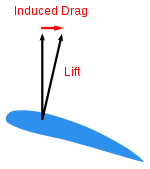Induced drag vs. lift

Lift-induced drag (also called induced drag) is drag which occurs as the result of the creation of lift on a three-dimensional lifting body, such as the wing or fuselage of an airplane. Induced drag consists of two primary components, including drag due to the creation of vortices (vortex drag) and the presence of additional viscous drag (lift-induced viscous drag). The vortices in the flow-field, present in the wake of a lifting body, derive from the turbulent mixing of air of varying pressure on the upper and lower surfaces of the body, which is a necessary condition for the creation of lift.

With other parameters remaining the same, as the lift generated by a body increases, so does the lift-induced drag. For an aircraft in flight, this means that as the angle of attack, and therefore the lift coefficient, increases to the point of stall, so does the lift-induced drag. At the onset of stall, lift is abruptly decreased, as is lift-induced drag, but viscous pressure drag, a component of parasite drag, increases due to the formation of turbulent unattached flow on the surface of the body.

### Parasitic drag

Parasitic drag (also called parasite drag) is drag caused by moving a solid object through a fluid. Parasitic drag is made up of multiple components including viscous pressure drag (form drag), and drag due to surface roughness (skin friction drag). Additionally, the presence of multiple bodies in relative proximity may incur so called interference drag, which is sometimes described as a component of parasitic drag.

In aviation, induced drag tends to be greater at lower speeds because a high angle of attack is required to maintain lift, creating more drag. However, as speed increases the induced drag becomes much less, but parasitic drag increases because the fluid is flowing faster around protruding objects increasing friction or drag. At even higher speeds in the transonic, wave drag enters the picture. Each of these forms of drag changes in proportion to the others based on speed. The combined overall drag curve therefore shows a minimum at some airspeed - an aircraft flying at this speed will be at or close to its optimal efficiency. Pilots will use this speed to maximize endurance (minimum fuel consumption), or maximise gliding range in the event of an engine failure.

### Power curve in aviation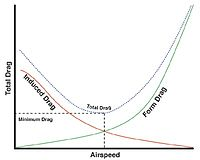The power curve: form and induced drag vs. airspeed

The interaction of parasitic and induced drag vs. airspeed can be plotted as a characteristic curve, illustrated here. In aviation, this is often referred to as the power curve, and is important to pilots because it shows that, below a certain airspeed, maintaining airspeed counterintuitively requires more thrust as speed decreases, rather than less. The consequences of being "behind the curve" in flight are important and are taught as part of pilot training. At the subsonic airspeeds where the "U" shape of this curve is significant, wave drag has not yet become a factor, and so it is not shown in the curve.

### Wave drag in transonic and supersonic flow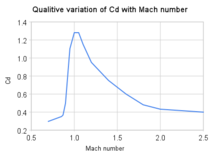Qualitative variation in Cd factor with Mach number for aircraft

Wave drag (also called compressibility drag) is drag which is created by the presence of a body moving at high speed through a compressible fluid. In aerodynamics, Wave drag consists of multiple components depending on the speed regime of the flight.

In transonic flight (Mach numbers greater than about 0.8 and less than about 1.4), wave drag is the result of the formation of shockwaves on the body, formed when areas of local supersonic (Mach number greater than 1.0) flow are created. In practice, supersonic flow occurs on bodies traveling well below the speed of sound, as the local speed of air on a body increases when it accelerates over the body, in this case above Mach 1.0. However, full supersonic flow over the vehicle will not develop until well past Mach 1.0. Aircraft flying at transonic speed often incur wave drag through the normal course of operation. In transonic flight, wave drag is commonly referred to as transonic compressibility drag. Transonic compressibility drag increases significantly as the speed of flight increases towards Mach 1.0, dominating other forms of drag at these speeds.

In supersonic flight (Mach numbers greater than 1.0), wave drag is the result of shockwaves present on the body, typically oblique shockwaves formed at the leading and trailing edges of the body. In highly supersonic flows, or in bodies with turning angles sufficiently large, unattached shockwaves, or bow waves will instead form. Additionally, local areas of transonic flow behind the initial shockwave may occur at lower supersonic speeds, and can lead to the development of additional, smaller shockwaves present on the surfaces of other lifting bodies, similar to those found in transonic flows. In supersonic flow regimes, wave drag is commonly separated into two components, supersonic lift-dependent wave drag and supersonic volume-dependent wave drag.

The closed form solution for the minimum wave drag of a body of revolution with a fixed length was found by Sears and Haack, and is known as the Sears-Haack Distribution. Similarly, for a fixed volume, the shape for minimum wave drag is the Von Karman Ogive.

Busemann's Biplane is not, in principle, subject to wave drag at all when operated at its design speed, but is incapable of generating lift.

In 1752 d'Alembert proved that potential flow, the 18th century state-of-the-art inviscid flow theory amenable to mathematical solutions, resulted in the prediction of zero drag. This was in contradiction with experimental evidence, and became known as d'Alembert's paradox. In the 19th century the Navier–Stokes equations for the description of viscous flow were developed by Saint-Venant, Navier and Stokes. Stokes derived the drag around a sphere at very low Reynolds numbers, the result of which is called Stokes law.

In the limit of high-Reynolds numbers the Navier–Stokes equations approach the inviscid Euler equations; of which the potential-flow solutions considered by d'Alembert are solutions. However, at high Reynolds numbers all experiments showed there is drag. Attempts to construct inviscid steady flow solutions to the Euler equations, other than the potential flow solutions, did not result in realistic results.

The notion of boundary layers—introduced by Prandtl in 1904, founded on both theory and experiments—explained the causes of drag at high Reynolds numbers. The boundary layer is the thin layer of fluid close to the object's boundary, where viscous effects remain important when the viscosity becomes very small (or equivalently the Reynolds number becomes very large).

Wikimedia Foundation. 2010.

Поможем написать реферат

### Look at other dictionaries:

• Drag — Contents 1 In science and technology 2 In arts, performance arts and entertainment 3 Other uses …   Wikipedia

• Drag equation — In fluid dynamics, the drag equation is a practical formula used to calculate the force of drag experienced by an object due to movement through a fully enclosing fluid. The equation is attributed to Lord Rayleigh, who originally used L2 in place …   Wikipedia

• drag — I UK [dræɡ] / US verb Word forms drag : present tense I/you/we/they drag he/she/it drags present participle dragging past tense dragged past participle dragged ** 1) [transitive] to pull something or someone along with difficulty, for example… …   English dictionary

• Drag crisis — In fluid dynamics, drag crisis is a phenomenon in which drag coefficient drops off suddenly as Reynolds number increases. This has been well studied for round bodies like spheres and cylinders. The drag coefficient of a sphere will change rapidly …   Wikipedia

• Physics of flying discs — A flying disc can fly through the air because of its shape, weight, initial direction of throw, and spin. The successful flight of a particular disc is determined by these variables as well as others such as deformation.uitability of a discA disc …   Wikipedia

• Automobile drag coefficient — The drag coefficient is a common metric in automotive design, where designers strive to achieve a low coefficient. Minimizing drag is done to improve fuel efficiency at highway speeds, where aerodynamic effects represent a substantial fraction of …   Wikipedia

• Very-low-drag bullet — Very low drag bullets (VLD) are primarily a small arms ballistics development of the 1980s–1990s, driven by shooters desire for bullets that will give a higher degree of accuracy and kinetic efficiency, especially at extended ranges. To achieve… …   Wikipedia

• form drag — Physics. the portion of the resisting force encountered by a body moving through a fluid that is due to the irregularity of shape of the body, reducible to a minimum by streamlining. [1930 35] * * * n. Aeronautics that part of the drag on an… …   Useful english dictionary

• form drag — Physics. the portion of the resisting force encountered by a body moving through a fluid that is due to the irregularity of shape of the body, reducible to a minimum by streamlining. [1930 35] * * * …   Universalium

• Aether drag hypothesis — In the 19th century, the theory of the luminiferous aether as the hypothetical medium for the propagation of light, was widely discussed. An important part of this discussion was the question concerning the state of motion of Earth with respect… …   Wikipedia